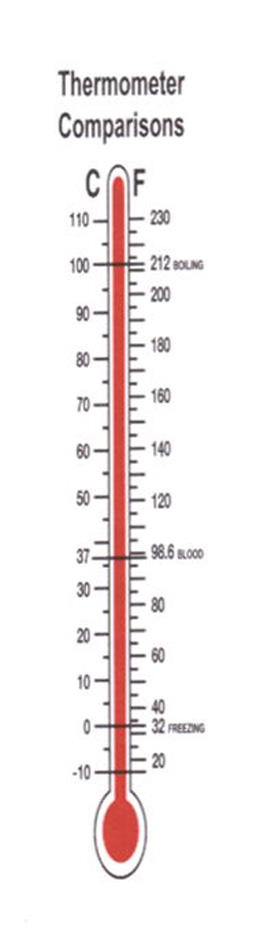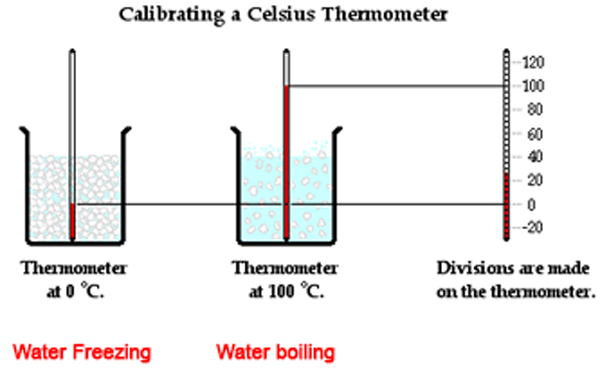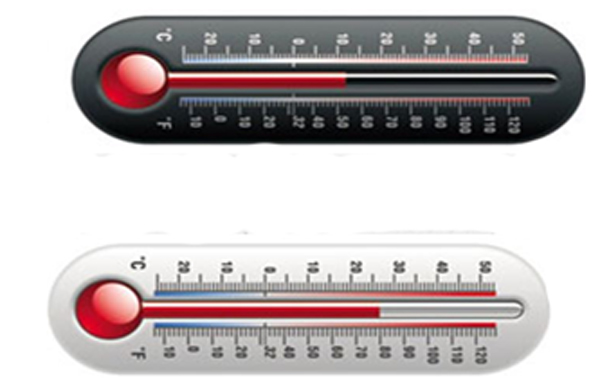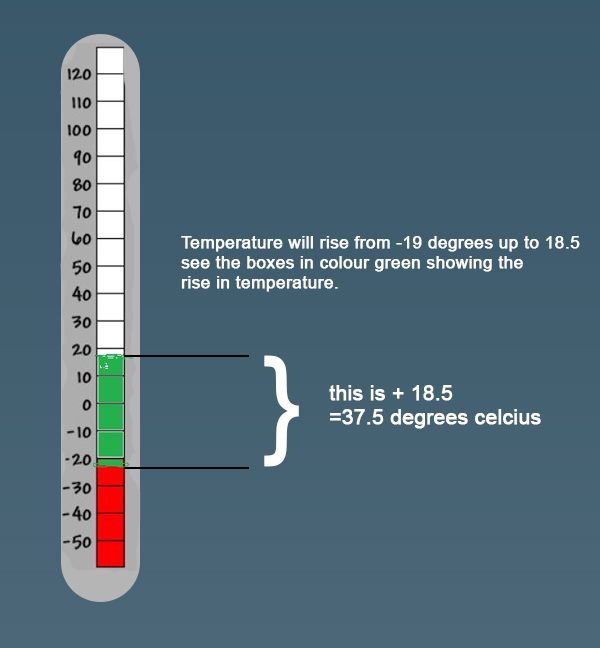Temperature

Temperature measures how hot or cold a thing is. Temperature is measured using a thermometer, usually in the Celsius or Fahrenheit scale.

Below is an image of a thermometer.Thermometer readings can be recorded and studied so that we can see the rise and fall in temperature.

We can also work out the temperature at a given time of the temperature change at the same rate.

At the sea level, for example at Mombasa, water boils at 100 degree celcius and freezes at 0 degrees celcius.

#### Look at this imagePlay the video below to learn  how to read a thermometer

The diagrams below show different thermometers. Read and record the temperature shown#### Solution

a. 12 degrees celcius

b. 26 degrees celcius

The reading on the thermometer used in patients shows -19 oc before it is used.

After being placed under the armpit, the mercury rose to 18.5 oc.

What was the temperature of the body?

####One day Adan studied the changes in room temperature by taking the readings of a thermometer from 6.00 a.m to 2.00 p.m the temperature changed from 18 0c to 340c.

At 6.00 p.m the temperature was 24 0c.

Work out:

(a) Difference in temperature between 2.00 p.m and 6.00 p.m.

(b) Temperature at 11.00 p.m if the temperature rose at the same rate.

(c) Temperature at 4.00 p.m if the fall in temperature was at the same rate.

Solution

a) Temperature at 2pm was at was 34 degrees celcius

Temperature at 6 p.m was 24 degrees celcius

Difference in temperature was 34 - 24 = 10  degrees celcius

b) Change in temperature is

34 - 18 = 16 degrees celcius

Time between 6.00 a.m and 2 p.m = 8 h

Rise in temperature per hour =

change in temperature divided by time taken

16 degrees celcius / 8 h

Time between 6.00am and 11 am = 5 h

Temperature at 11.00a.m was

18 degrees celcius + (2 x 5) degrees celcius = 28 degrees celcius

c) change in temperature between 2.00 p.m and 6.00 p.m is

34 degrees celcius - 24 degrees celcius = 10 degrees celcius

Time between 2.00 p.m and 6.00 p.m is 4 h

Fall in temperature per hour =

change in temperature divide by time taken

= 10 degrees celcius / 4 h

= 2.5degrees celcius per hour

Time between 2.00 p.m and 4.00 p.m = 2 h

Temperature at 4 p.m is

34 degrees celcius - (2.5 x 2) degrees celcius

= 29 degrees celcius